• 神经网络学习心得
千次阅读
2019-10-19 18:58:15

人工智能（AI）从诞生以来，理论和技术日益成熟，应用领域也不断扩大。目前人工智能（AI）已经以雷霆万钧之势从学术界潜入产业界的每一个角落，其价值之大，可想而知。人工智能是一门极富挑战性的科学，从事这项工作的人必须懂得计算机知识，心理学和哲学。机器学习中的神经网络是研究人工智能最热门的方向，而多层复杂的神经网络又发展成现在的深度学习。

1. 数学基础：高等数学、线性代数、概率论与数理统计（后面两个在本文中可能显得不是那么重要）
2. 编程基础：Python（https://www.runoob.com/python/python-tutorial.html

1.AI从入门到放弃：BP神经网络算法推导及代码实现笔记：https://zhuanlan.zhihu.com/p/38006693

总结：该部分是学习神经网络的前提，其中梯度下降法需要重点掌握（用到高数中不少知识点），神经网络中的梯度下降法或BP算法用到了高数中的链式求导法则（可以跟着这个方向走）。链接的内容很多，但是需要我们掌握的有三点，梯度下降法、BP算法、loss损失函数的自定义（提供的链接没有提到，但是用到了，自定义损失函数有些人用到了信息论中的知识）。

总结：自认为讲得还不错，自己也用了pytorch的框架。但是也有必要列出其他神经网络框架，如下图所示。pytorch框架学习教程：https://github.com/zergtant/pytorch-handbook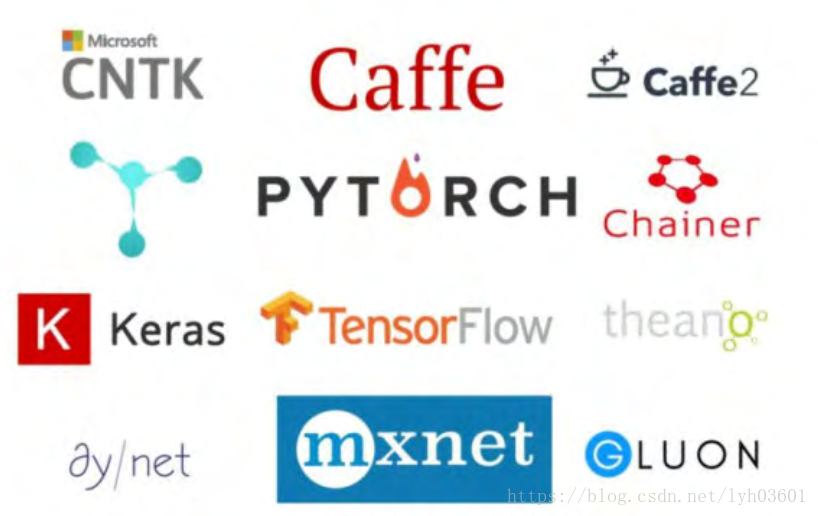3.搭建我们所需的环境：这部分我也弄了很长时间，主要原因是pytorch所需要的包都是从国外下载，下载速度很慢导致崩溃。

第一步，选择Python的编程工具

推荐使用清华源anaconda（https://mirrors.tuna.tsinghua.edu.cn/anaconda/archive/），下载好后安装即可，该工具自带Python环境。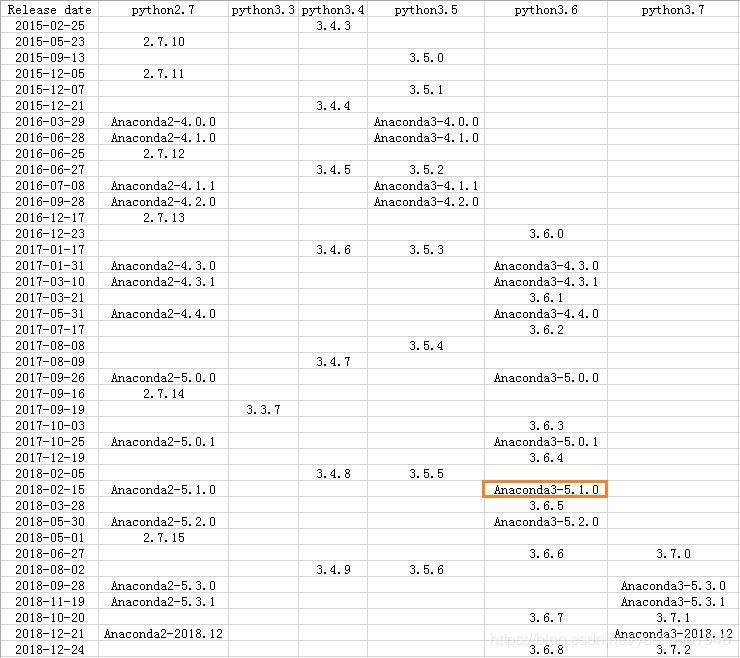第二步，安装pytorch环境

注意：第二步安装很容易出错。

所有工作完成好后，我们就可以在GitHub（https://github.com/）找个项目来跑跑了（本文不作详细的说明了）。跑完之后就可以自己尝试着写代码了。最后，放上几个感觉还不错的链接，因为写本文的初衷就是想收藏几个链接，生怕以后找不到了。

卷积神经网络特征图可视化（自定义网络和VGG网络）：

https://blog.csdn.net/dcrmg/article/details/81255498

Pytorch中正确设计并加载数据集方法：

https://ptorch.com/news/215.html

pytorch使用（一）处理并加载自己的数据：

https://blog.csdn.net/gyguo95/article/details/78821520/

Pytorch：从读取数据到项目落地：

https://blog.csdn.net/qq_26697045/article/details/100987856

Faster RCNN的TensorFlow代码的理解：

https://www.cnblogs.com/darkknightzh/p/10043864.html

faster-rcnn代码解读记录，github+tf：

https://blog.csdn.net/qq_41576083/article/details/82966489

更多相关内容
• 神经网络学习小记录52——Pytorch 搭建孪生神经网络比较图片相似性学习前言什么是孪生神经网络孪生神经网络的实现思路一、预测部分1、主干网络介绍2、比较网络二、训练部分1、数据集的格式2、Loss计算训练自己的孪生...

# 学习前言

也学习一下Pytorch版本的。# 什么是孪生神经网络

简单来说，孪生神经网络（Siamese network）就是“连体的神经网络”，神经网络的“连体”是通过共享权值来实现的，如下图所示。所谓权值共享就是当神经网络有两个输入的时候，这两个输入使用的神经网络的权值是共享的（可以理解为使用了同一个神经网络）。

很多时候，我们需要去评判两张图片的相似性，比如比较两张人脸的相似性，我们可以很自然的想到去提取这个图片的特征再进行比较，自然而然的，我们又可以想到利用神经网络进行特征提取
如果使用两个神经网络分别对图片进行特征提取，提取到的特征很有可能不在一个域中，此时我们可以考虑使用一个神经网络进行特征提取再进行比较。这个时候我们就可以理解孪生神经网络为什么要进行权值共享了。

孪生神经网络有两个输入（Input1 and Input2），利用神经网络将输入映射到新的空间，形成输入在新的空间中的表示。通过Loss的计算，评价两个输入的相似度。

# 代码下载

https://github.com/bubbliiiing/Siamese-pytorch

# 孪生神经网络的实现思路

## 一、预测部分

### 1、主干网络介绍孪生神经网络的主干特征提取网络的功能是进行特征提取，各种神经网络都可以适用，本文使用的神经网络是VGG16。
关于VGG的介绍大家可以看我的另外一篇博客https://blog.csdn.net/weixin_44791964/article/details/102779878这是一个VGG被用到烂的图，但确实很好的反应了VGG的结构：
1、一张原始图片被resize到指定大小，本文使用105x105。
2、conv1包括两次[3,3]卷积网络，一次2X2最大池化，输出的特征层为64通道。
3、conv2包括两次[3,3]卷积网络，一次2X2最大池化，输出的特征层为128通道。
4、conv3包括三次[3,3]卷积网络，一次2X2最大池化，输出的特征层为256通道。
5、conv4包括三次[3,3]卷积网络，一次2X2最大池化，输出的特征层为512通道。
6、conv5包括三次[3,3]卷积网络，一次2X2最大池化，输出的特征层为512通道。

实现代码为：

import torch
import torch.nn as nn

class VGG(nn.Module):
def __init__(self, features, num_classes=1000):
super(VGG, self).__init__()
self.features = features
self.classifier = nn.Sequential(
nn.Linear(512 * 7 * 7, 4096),
nn.ReLU(True),
nn.Dropout(),
nn.Linear(4096, 4096),
nn.ReLU(True),
nn.Dropout(),
nn.Linear(4096, num_classes),
)
self._initialize_weights()

def forward(self, x):
x = self.features(x)
x = self.avgpool(x)
x = torch.flatten(x, 1)
x = self.classifier(x)
return x

def _initialize_weights(self):
for m in self.modules():
if isinstance(m, nn.Conv2d):
nn.init.kaiming_normal_(m.weight, mode='fan_out', nonlinearity='relu')
if m.bias is not None:
nn.init.constant_(m.bias, 0)
elif isinstance(m, nn.BatchNorm2d):
nn.init.constant_(m.weight, 1)
nn.init.constant_(m.bias, 0)
elif isinstance(m, nn.Linear):
nn.init.normal_(m.weight, 0, 0.01)
nn.init.constant_(m.bias, 0)

def make_layers(cfg, batch_norm=False):
layers = []
in_channels = 1
for v in cfg:
if v == 'M':
layers += [nn.MaxPool2d(kernel_size=2, stride=2)]
else:
conv2d = nn.Conv2d(in_channels, v, kernel_size=3, padding=1)
if batch_norm:
layers += [conv2d, nn.BatchNorm2d(v), nn.ReLU(inplace=True)]
else:
layers += [conv2d, nn.ReLU(inplace=True)]
in_channels = v
return nn.Sequential(*layers)

cfgs = {
'D': [64, 64, 'M', 128, 128, 'M', 256, 256, 256, 'M', 512, 512, 512, 'M', 512, 512, 512, 'M']
}

def VGG16(pretrained, **kwargs):
model = VGG(make_layers(cfgs["D"], batch_norm=False), **kwargs)
if pretrained:
return model


### 2、比较网络在获得主干特征提取网络之后，我们可以获取到一个多维特征，我们可以使用flatten的方式将其平铺到一维上，这个时候我们就可以获得两个输入的一维向量了

将这两个一维向量进行相减，再进行绝对值求和，相当于求取了两个特征向量插值的L1范数。也就相当于求取了两个一维向量的距离。

然后对这个距离再进行两次全连接，第二次全连接到一个神经元上，对这个神经元的结果取sigmoid，使其值在0-1之间，代表两个输入图片的相似程度。

实现代码如下：

import torch
import torch.nn as nn
from nets.vgg import VGG16

def get_img_output_length(width, height):
def get_output_length(input_length):
# input_length += 6
filter_sizes = [2, 2, 2, 2, 2]
padding = [0, 0, 0, 0, 0]
stride = 2
for i in range(5):
input_length = (input_length+2*padding[i]-filter_sizes[i]) // stride + 1
return input_length
return get_output_length(width)*get_output_length(height)

class Siamese(nn.Module):
def __init__(self, input_shape, pretrained=False):
super(Siamese, self).__init__()
self.vgg = VGG16(pretrained)
del self.vgg.avgpool
del self.vgg.classifier

flat_shape = 512 * get_img_output_length(input_shape,input_shape)
self.fully_connect1 = torch.nn.Linear(flat_shape,512)
self.fully_connect2 = torch.nn.Linear(512,1)

def forward(self, x):
x1, x2 = x
x1 = self.vgg.features(x1)
x2 = self.vgg.features(x2)
b, _, _, _ = x1.size()
x1 = x1.view([b,-1])
x2 = x2.view([b,-1])
x = torch.abs(x1-x2)
x = self.fully_connect1(x)
x = self.fully_connect2(x)
return x


## 二、训练部分

### 1、数据集的格式

本文所使用的数据集为Omniglot数据集。
其包含来自 50不同字母（语言）的1623 个不同手写字符。每一个字符都是由 20个不同的人通过亚马逊的 Mechanical Turk 在线绘制的。

相当于每一个字符有20张图片，然后存在1623个不同的手写字符，我们需要利用神经网络进行学习，去区分这1623个不同的手写字符，比较输入进来的字符的相似性。

本博客中数据存放格式有三级：

- image_background
- Alphabet_of_the_Magi
- character01
- 0709_01.png
- 0709_02.png
- ……
- character02
- character03
- ……
- Anglo-Saxon_Futhorc
- ……


最后一级的文件夹用于分辨不同的字体，同一个文件夹里面的图片属于同一文字。在不同文件夹里面存放的图片属于不同文字。上两个图为.\images_background\Alphabet_of_the_Magi\character01里的两幅图。它们两个属于同一个字。上一个图为.\images_background\Alphabet_of_the_Magi\character02里的一幅图。它和上面另两幅图不属于同一个字。

### 2、Loss计算

对于孪生神经网络而言，其具有两个输入。

当两个输入指向同一个类型的图片时，此时标签为1。

当两个输入指向不同类型的图片时，此时标签为0。

然后将网络的输出结果和真实标签进行交叉熵运算，就可以作为最终的loss了。

本文所使用的Loss为binary_crossentropy。

当我们输入如下两个字体的时候，我们希望网络的输出为1。我们会将预测结果和1求交叉熵。

当我们输入如下两个字体的时候，我们希望网络的输出为0。我们会将预测结果和0求交叉熵。

# 训练自己的孪生神经网络

## 1、训练本文所使用的Omniglot例子下载数据集，放在根目录下的dataset文件夹下。运行train.py开始训练。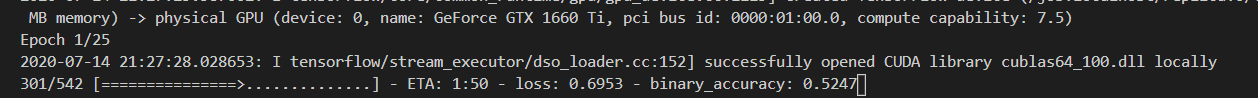## 2、训练自己相似性比较的模型

如果大家想要训练自己的数据集，可以将数据集按照如下格式进行摆放。每一个chapter里面放同类型的图片。
之后将train.py当中的train_own_data设置成True，即可开始训练。展开全文图片相似性
• 神经网络学习小记录51——Keras 搭建孪生神经网络比较图片相似性学习前言什么是孪生神经网络孪生神经网络的实现思路一、预测部分1、主干网络介绍 学习前言 最近学习了一下如何比较两张图片的相似性，用到了孪生神经...

# 学习前言

最近学习了一下如何比较两张图片的相似性，用到了孪生神经网络，一起来学习一下。# 什么是孪生神经网络

简单来说，孪生神经网络（Siamese network）就是“连体的神经网络”，神经网络的“连体”是通过共享权值来实现的，如下图所示。所谓权值共享就是当神经网络有两个输入的时候，这两个输入使用的神经网络的权值是共享的（可以理解为使用了同一个神经网络）。

很多时候，我们需要去评判两张图片的相似性，比如比较两张人脸的相似性，我们可以很自然的想到去提取这个图片的特征再进行比较，自然而然的，我们又可以想到利用神经网络进行特征提取
如果使用两个神经网络分别对图片进行特征提取，提取到的特征很有可能不在一个域中，此时我们可以考虑使用一个神经网络进行特征提取再进行比较。这个时候我们就可以理解孪生神经网络为什么要进行权值共享了。

孪生神经网络有两个输入（Input1 and Input2），利用神经网络将输入映射到新的空间，形成输入在新的空间中的表示。通过Loss的计算，评价两个输入的相似度。

# 代码下载

https://github.com/bubbliiiing/Siamese-keras

# 孪生神经网络的实现思路

## 一、预测部分

### 1、主干网络介绍孪生神经网络的主干特征提取网络的功能是进行特征提取，各种神经网络都可以适用，本文使用的神经网络是VGG16。
关于VGG的介绍大家可以看我的另外一篇博客https://blog.csdn.net/weixin_44791964/article/details/102779878这是一个VGG被用到烂的图，但确实很好的反应了VGG的结构：
1、一张原始图片被resize到指定大小，本文使用105x105。
2、conv1包括两次[3,3]卷积网络，一次2X2最大池化，输出的特征层为64通道。
3、conv2包括两次[3,3]卷积网络，一次2X2最大池化，输出的特征层为128通道。
4、conv3包括三次[3,3]卷积网络，一次2X2最大池化，输出的特征层为256通道。
5、conv4包括三次[3,3]卷积网络，一次2X2最大池化，输出的特征层为512通道。
6、conv5包括三次[3,3]卷积网络，一次2X2最大池化，输出的特征层为512通道。

实现代码为：

import keras
from keras.layers import Input,Dense,Conv2D
from keras.layers import MaxPooling2D,Flatten
from keras.models import Model
import os
import numpy as np
from PIL import Image
from keras.optimizers import SGD

class VGG16:
def __init__(self):
self.block1_conv1 = Conv2D(64,(3,3),activation = 'relu',padding = 'same',name = 'block1_conv1')
self.block1_conv2 = Conv2D(64,(3,3),activation = 'relu',padding = 'same', name = 'block1_conv2')
self.block1_pool = MaxPooling2D((2,2), strides = (2,2), name = 'block1_pool')

self.block2_conv1 = Conv2D(128,(3,3),activation = 'relu',padding = 'same',name = 'block2_conv1')
self.block2_conv2 = Conv2D(128,(3,3),activation = 'relu',padding = 'same',name = 'block2_conv2')
self.block2_pool = MaxPooling2D((2,2),strides = (2,2),name = 'block2_pool')

self.block3_conv1 = Conv2D(256,(3,3),activation = 'relu',padding = 'same',name = 'block3_conv1')
self.block3_conv2 = Conv2D(256,(3,3),activation = 'relu',padding = 'same',name = 'block3_conv2')
self.block3_conv3 = Conv2D(256,(3,3),activation = 'relu',padding = 'same',name = 'block3_conv3')
self.block3_pool = MaxPooling2D((2,2),strides = (2,2),name = 'block3_pool')

self.block4_conv1 = Conv2D(512,(3,3),activation = 'relu',padding = 'same', name = 'block4_conv1')
self.block4_conv2 = Conv2D(512,(3,3),activation = 'relu',padding = 'same', name = 'block4_conv2')
self.block4_conv3 = Conv2D(512,(3,3),activation = 'relu',padding = 'same', name = 'block4_conv3')
self.block4_pool = MaxPooling2D((2,2),strides = (2,2),name = 'block4_pool')

# 第五个卷积部分
self.block5_conv1 = Conv2D(512,(3,3),activation = 'relu',padding = 'same', name = 'block5_conv1')
self.block5_conv2 = Conv2D(512,(3,3),activation = 'relu',padding = 'same', name = 'block5_conv2')
self.block5_conv3 = Conv2D(512,(3,3),activation = 'relu',padding = 'same', name = 'block5_conv3')
self.block5_pool = MaxPooling2D((2,2),strides = (2,2),name = 'block5_pool')

self.flatten = Flatten(name = 'flatten')

def call(self, inputs):
x = inputs
x = self.block1_conv1(x)
x = self.block1_conv2(x)
x = self.block1_pool(x)

x = self.block2_conv1(x)
x = self.block2_conv2(x)
x = self.block2_pool(x)

x = self.block3_conv1(x)
x = self.block3_conv2(x)
x = self.block3_conv3(x)
x = self.block3_pool(x)

x = self.block4_conv1(x)
x = self.block4_conv2(x)
x = self.block4_conv3(x)
x = self.block4_pool(x)

x = self.block5_conv1(x)
x = self.block5_conv2(x)
x = self.block5_conv3(x)
x = self.block5_pool(x)

outputs = self.flatten(x)
return outputs


### 2、比较网络在获得主干特征提取网络之后，我们可以获取到一个多维特征，我们可以使用flatten的方式将其平铺到一维上，这个时候我们就可以获得两个输入的一维向量了

将这两个一维向量进行相减，再进行绝对值求和，相当于求取了两个特征向量插值的L1范数。也就相当于求取了两个一维向量的距离。

然后对这个距离再进行两次全连接，第二次全连接到一个神经元上，对这个神经元的结果取sigmoid，使其值在0-1之间，代表两个输入图片的相似程度。

实现代码如下：

import keras
from keras.layers import Input,Dense,Conv2D
from keras.layers import MaxPooling2D,Flatten,Lambda
from keras.models import Model
import keras.backend as K
import os
import numpy as np
from PIL import Image
from keras.optimizers import SGD
from nets.vgg import VGG16

def siamese(input_shape):
vgg_model = VGG16()

input_image_1 = Input(shape=input_shape)
input_image_2 = Input(shape=input_shape)

encoded_image_1 = vgg_model.call(input_image_1)
encoded_image_2 = vgg_model.call(input_image_2)

l1_distance_layer = Lambda(
lambda tensors: K.abs(tensors - tensors))
l1_distance = l1_distance_layer([encoded_image_1, encoded_image_2])

out = Dense(512,activation='relu')(l1_distance)
out = Dense(1,activation='sigmoid')(out)

model = Model([input_image_1,input_image_2],out)
return model


## 二、训练部分

### 1、数据集的格式

本文所使用的数据集为Omniglot数据集。
其包含来自 50不同字母（语言）的1623 个不同手写字符。每一个字符都是由 20个不同的人通过亚马逊的 Mechanical Turk 在线绘制的。

相当于每一个字符有20张图片，然后存在1623个不同的手写字符，我们需要利用神经网络进行学习，去区分这1623个不同的手写字符，比较输入进来的字符的相似性。

本博客中数据存放格式有三级：

- image_background
- Alphabet_of_the_Magi
- character01
- 0709_01.png
- 0709_02.png
- ……
- character02
- character03
- ……
- Anglo-Saxon_Futhorc
- ……


最后一级的文件夹用于分辨不同的字体，同一个文件夹里面的图片属于同一文字。在不同文件夹里面存放的图片属于不同文字。上两个图为.\images_background\Alphabet_of_the_Magi\character01里的两幅图。它们两个属于同一个字。上一个图为.\images_background\Alphabet_of_the_Magi\character02里的一幅图。它和上面另两幅图不属于同一个字。

### 2、Loss计算

对于孪生神经网络而言，其具有两个输入。

当两个输入指向同一个类型的图片时，此时标签为1。

当两个输入指向不同类型的图片时，此时标签为0。

然后将网络的输出结果和真实标签进行交叉熵运算，就可以作为最终的loss了。

本文所使用的Loss为binary_crossentropy。

当我们输入如下两个字体的时候，我们希望网络的输出为1。我们会将预测结果和1求交叉熵。

当我们输入如下两个字体的时候，我们希望网络的输出为0。我们会将预测结果和0求交叉熵。

# 训练自己的孪生神经网络

## 1、训练本文所使用的Omniglot例子下载数据集，放在根目录下的dataset文件夹下。运行train.py开始训练。## 2、训练自己相似性比较的模型

如果大家想要训练自己的数据集，可以将数据集按照如下格式进行摆放。每一个chapter里面放同类型的图片。
之后将train.py当中的train_own_data设置成True，即可开始训练。展开全文Siamese keras
• BP神经网络算法是在BP神经网络现有算法的基础上提出的，是通过任意选定一组权值，将给定的目标输出直接作为线性方程的代数和来建立线性方程组，解得待求权，不存在传统方法的局部极小及收敛速度慢的问题，且更易理解...

## 一、 由Multi-layer Feedforward Neural Network到Back Propagation

### 1.1 多层前馈神经网络

在文章ANN人工神经网络(Artificial Neuron Network )已经介绍了从B-P神经元模型到多层感知机模型。其中的多层感知机模型，又叫多层神经网络，是多层前馈神经网络（Multi-layer Feedforward Neural Network：MLFNN）中的一种。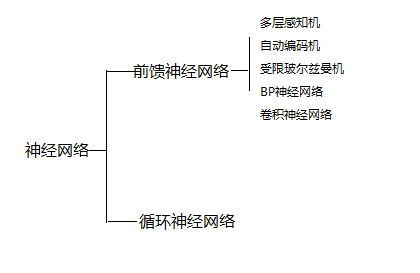多层神经网络的拓扑结构如下图所示：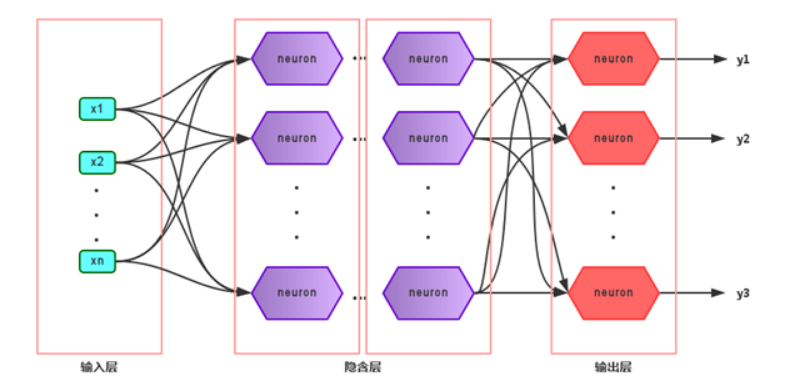在神经网络中，输入层与输出层之间的层称为隐含层或隐层（hidden layer），隐层和输出层的神经元都是具有激活函数的功能神经元。 只需包含一个隐层便可以称为多层神经网络，常用的神经网络称为“多层前馈神经网络”（multi-layer feedforward neural network）这里的层实际上是隐含层的个数），该结构满足以下几个特点：

• 每层神经元与下一层神经元之间完全互连

• 神经元之间不存在同层连接

• 神经元之间不存在跨层连接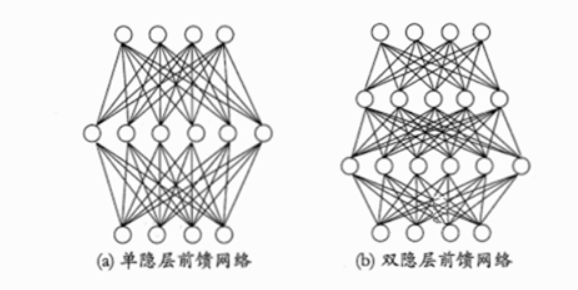注意：根据上面的特点可以得知：这里的“前馈”指的是网络拓扑结构中不存在环或回路，而不是指该网络只能向前传播而不能向后传播

### 1.2 逆向误差神经网络

这样，BP（Back Propagation）网络，实际上，也是是多层感知器的一种，是1986年由Rumelhart和Hinton为首的科学家小组提出，相比于多层感知机，BP网络除了具有前馈神经网络的是一种按误差逆传播算法训练的多层前馈网络，是目前应用最广泛、最成功的神经网络模型之一。BP网络能学习和存贮大量的输入-输出模式映射关系，而无需事前揭示描述这种映射关系的数学方程。它的学习规则是使用最速下降法，通过反向传播来不断调整网络的权值和阈值，使网络的误差平方和最小。BP神经网络模型拓扑结构包括输入层（input）、隐层(hide layer)和输出层(output layer)。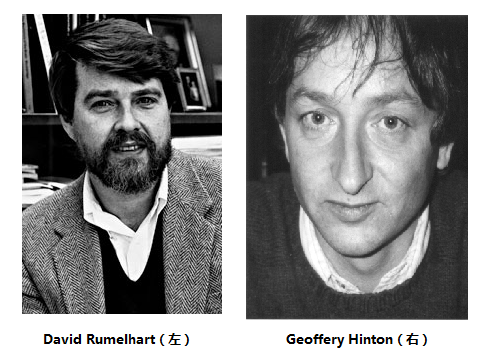BP神经网络是一种多层的前馈神经网络，其主要的特点是：信号是前向传播的，而误差是反向传播的。
举个例子：我要追求女神，那我总得表示一下吧！于是我给她买花，讨她欢心。然后，她给我一些表示（或者叫暗示），根据这个表示，与我最终目的进行对比（追求到女神），然后我进行调整继续表示，一直循环往复，知道实现最终目的——成功最求到女神。我的表示就是“信号前向传播”，女神的表示就是“误差反向传播”。这就是BP神经网络的核心。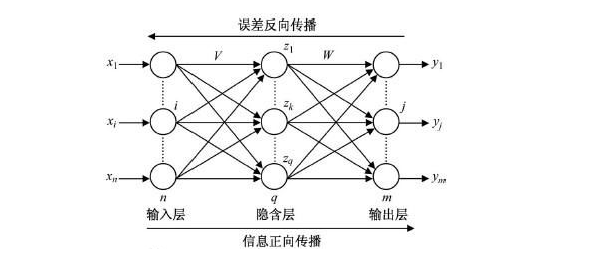3层BP神经网络

具体来说，对于如下的只含一个隐层的神经网络模型：BP神经网络的过程主要分为两个阶段，第一阶段是信号的前向传播，从输入层经过隐含层，最后到达输出层；第二阶段是误差的反向传播，从输出层到隐含层，最后到输入层，依次调节隐含层到输出层的权重和偏置，输入层到隐含层的权重和偏置。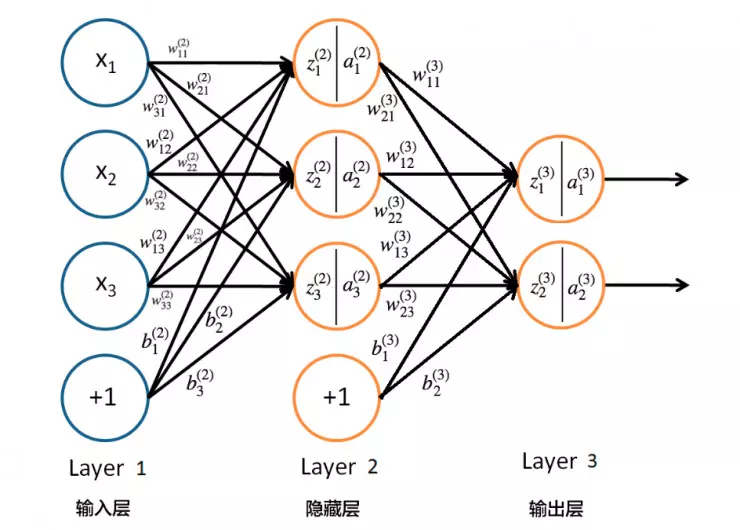3层BP神经网络

神经网络利用现有的数据找出输入与输出之间得权值关系（近似），然后利用这样的权值关系进行仿真，例如输入一组数据仿真出输出结果，当然你的输入要和训练时采用的数据集在一个范畴之内。例如预报天气：温度 湿度 气压等作为输入 天气情况作为输出利用历史得输入输出关系训练出神经网络，然后利用这样的神经网络输入今天的温度 湿度 气压等 得出即将得天气情况。同理，运用到自动化测试中，使用测试数据反映结果走向，bug数，质量问题等情况也可以做到提前预测的！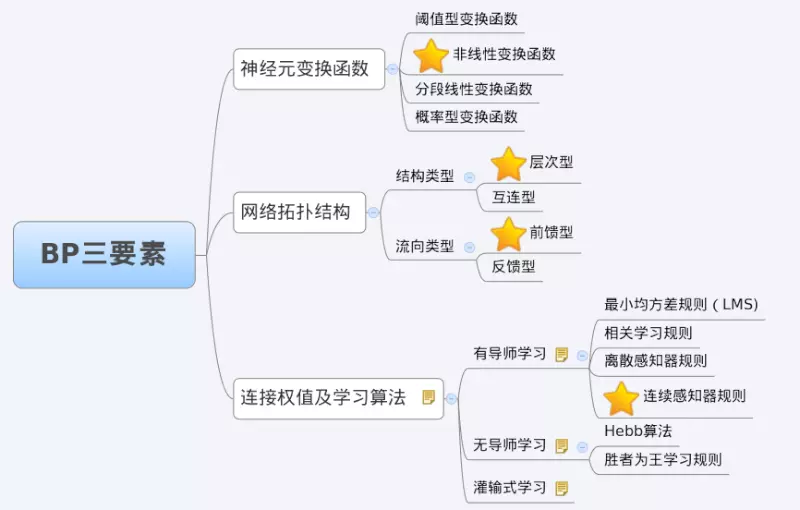## 二、 BP神经网络算法详解

### 2.1 BP网络使用的激活函数Sigmoid

BP网络采用的传递函数是非线性变换函数——Sigmoid函数（又称S函数）。其特点是函数本身及其导数都是连续的，因而在处理上十分方便。为什么要选择这个函数，等下在介绍BP网络的学习算法的时候会进行进一步的介绍。S函数有单极性S型函数和双极性S型函数两种，单极性S型函数定义如下： f ( x ) = 1 1 + e − x f(x)=\cfrac{1}{1+e^{-x}} 其函数曲线如图所示：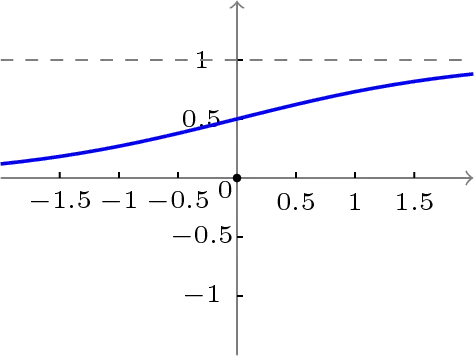单极性S曲线

双极性S型函数： f ( x ) = 1 − e − x 1 + e − x f(x)=\cfrac{1-e^{-x}}{1+e^{-x}}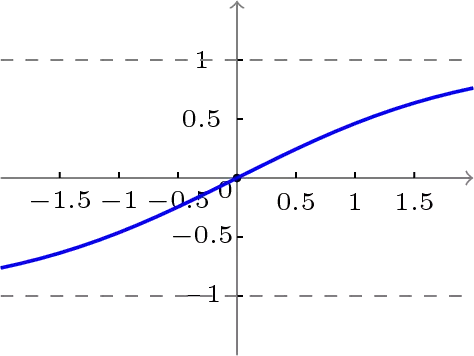双极性S曲线

使用S型激活函数时，输入：net = x 1 ω 1 x_1\omega_1 + x 2 ω 2 x_2\omega_2 + x 3 ω 3 x_3\omega_3 +…+ x n ω n x_n\omega_n
输出为：y = f(net) = 1 1 + e − n e t \cfrac{1}{1+e^{-net}}
输出的导数： f ’ ( n e t ) f^’(net) = 1 1 + e − n e t \cfrac{1}{1+e^{-net}} - 1 ( 1 + e − n e t ) 2 \cfrac{1}{(1+{e^{-net})}^2} = y(y-1)
使用S型激活函数时，BP网络的输出及其导数图形：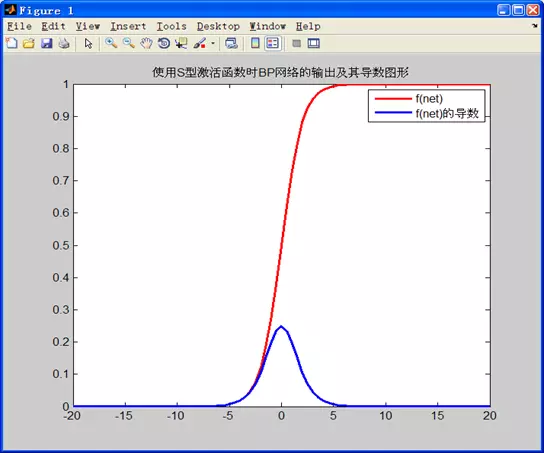根据S激活函数的图形：

• net在 -5~0 的时候导数的值为正，且导数的值逐渐增大，说明此时f(x)在逐渐变大且变大的速度越来越快
• net在 0~5 的时候导数的值为正，且导数的值逐渐减小，说明此时f(x)在逐渐变大但是变大的速度越来越慢
• 对神经网络进行训练，我们应该尽量将net的值尽量控制在收敛比较快的范围内。

### 2.2 BP算法如何进行误差反馈

#### 2.2.1 三层 BP 神经网络

反向传播算法,即Back Propagation是建立在梯度下降算法基础上，适用多层神经网络的参数训练方法。由于隐藏层节点的预测误差无法直接计算,因此,反向传播算法直接利用输出层节点的预测误差反向估计上一层隐藏节点的预测误差,即从后往前逐层从输出层把误差反向传播到输入层,从而实现对链接权重调整,这也是反向传播算法名称的由来。
BP (Back Propagation)神经网络，即误差反传误差反向传播算法的学习过程，由信息的正向传播和误差的反向传播两个过程组成。

• 输入层各神经元负责接收来自外界的输入信息，并传递给中间层各神经元;

• 中间层是内部信息处理层，负责信息变换，根据信息变化能力的需求，中间层可以设计为单隐层或者多隐层结构;其中隐藏层的节点个数经验公式为：S = 1 + [ m ∗ ( n + 2 ) ] 0.5 {1 + [m*(n+2)]}^{0.5} 其中S为隐藏层的节点个数，m为输入节点数，n为输出 节点数。

• 最后一个隐层传递到输出层各神经元的信息，经进一步处理后，完成一次学习的正向传播处理过程，由输出层向外界输出信息处理结果。

• 当实际输出与期望输出不符时，进入误差的反向传播阶段。误差通过输出层，按误差梯度下降的方式修正各层权值，向隐层、输入层逐层反传。周而复始的信息正向传播和误差反向传播过程，是各层权值不断调整的过程，也是神经网络学习训练的过程，此过程一直进行到网络输出的误差减少到可以接受的程度，或者预先设定的学习次数为止。
一个典型的3层BP神经网络模型如下图所示: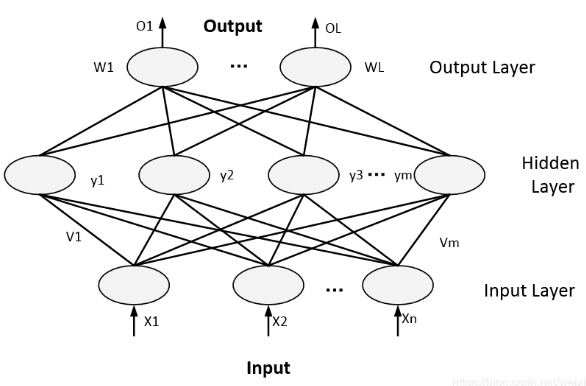三层BP神经网络示意图

如上图所示的三层神经网络中，W=（W1，W2,…,Wj,…,Wl）,其中列向量Wj=(Wj1，Wj2,…,Wjm)表示输出层神经元j与隐藏层神经元k的连接权重。
对于神经网络输出层有：

O k O_k = f( n e t k net_k )                                    (1)
n e t k net_k = ∑ j = 0 m ω f k y k \sum_{j=0}^m\omega_{fk} y_{k} ,k=1,2,…,l                      (2)

对于神经网络隐藏层有：

y j y_j = f( n e t j net_j ),j = 1,2,…,m                             (3)
n e t j net_j = ∑ r = 0 n ν r f χ r \sum_{r=0}^n\nu_{rf} \chi_{r} ,k=1,2,…,l                          (4)

在上面的两个式子中的激活函数(或转移函数)根据应用需要可以均为Sigmoid函数，或者均为双极S形函数。以上式(1)~( 4 )以及激活函数共同构成了三层前馈网的数学模型。

#### 2.2.2 网络误差与权值调整

当神经网络的输出与期望的输出差距较大或者不在接受范围内时，即存在输出误差，其定义如下：

E = 1 2 \cfrac{1}{2} . ( d − O ) 2 (d-O)^2 = 1 2 \cfrac{1}{2} . ∑ k = 1 l ( d k − O k ) 2 \sum_{k=1}^l(d_k-O_k)^2                   (5)

为什么要乘以 1 2 \cfrac{1}{2} 的原因是，E求导后系数会为1。 将上面的误差定义表达式展开，带入隐藏层数据有：

E = 1 2 \cfrac{1}{2} . ∑ k = 1 l [ d k − f ( n e t k ) ] 2 \sum_{k=1}^l[d_k-f(net_k)]^2                        (6)

表达式展开到输入层，则有：

E = 1 2 \cfrac{1}{2} . ∑ k = 1 l ( d k − f [ ∑ r = 0 m w r k f ( n e t r ) ] ) 2 \sum_{k=1}^l({d_k-f[\sum_{r=0}^m w_{rk}f(net_r)]})^2
= 1 2 \cfrac{1}{2} . ∑ k = 1 l ( d k − f [ ∑ r = 0 m w r k f ( ∑ r = 0 n ν r f χ r ) ] ) 2 \sum_{k=1}^l({d_k-f[\sum_{r=0}^m w_{rk}f(\sum_{r=0}^n\nu_{rf} \chi_{r} )]})^2                (7)

根据上式可以看出，神经网络输出的误差E是关于各层权值的函数，因此可以通过调整各层的权值来改变误差E的大小。显然，进行权值调整的目的是不断减少误差E的大小，使其符合具体的要求。因此，要求权值的调整量与误差的负梯度成正比，即:

Δ \Delta ω j k \omega_{jk} = - η ∂ E ∂ ω j k \eta\frac{\partial E}{\partial \omega_{jk}} ,j = 0,1,2,…,m;k = 1,2,…,l                (8.a)
Δ \Delta ν r f \nu_{rf} = - η ∂ E ∂ ν r f \eta\frac{\partial E}{\partial \nu_{rf}} ,r = 0,1,2,…,n;j = 1,2,…,m                (8.b)

上式中，负号表示梯度下降，比例系数用常数表示，它就是神经网络训练中的学习速率。

#### 2.2.3 BP算法推导

式(8)给出的是权值调整思路的数学表达，而并非神经网络权值调整的具体计算公式，下面经给出三层BP神经网络权值的具体计算公式。
对于BP神经网络输出层，式(8.a)可以写为:
Δ \Delta ω j k \omega_{jk} = - η ∂ E ∂ ω j k \eta\frac{\partial E}{\partial \omega_{jk}} = - η ∂ E ∂ n e t k ∂ n e t k ∂ ω j k \eta\frac{\partial E}{\partial net_{k}}\frac{\partial net_{k}}{\partial \omega_{jk}}                                        (9.a)

式(8.b)可以写为:

Δ \Delta ν r f \nu_{rf} = - η ∂ E ∂ n e t j ∂ n e t j ∂ ν r f \eta\frac{\partial E}{\partial net_{j}}\frac{\partial net_{j}}{\partial \nu_{rf}}                       (9.b)

定义输出层和隐藏层信号分别为：

δ k 0 \delta_k^0 = - η ∂ E ∂ n e t k \eta\frac{\partial E}{\partial net_{k}} δ j y \delta_j^y = - η ∂ E ∂ n e t j \eta\frac{\partial E}{\partial net_{j}}                             (10)

由（9.a）和（2）得：

Δ \Delta ω j k \omega_{jk} = η δ k 0 y j \eta \delta_k^0 y_j                                  (11.a)

由（9.b）和（4）得：

Δ \Delta ν r f \nu_{rf} = η δ j y x r \eta \delta_j^y x_r                         (11.b)

通过(11.a) 和(11.b)可以看出要完成神经网络权值调整计算的推到，只需要计算出式(10)中的误差信号，下面继续求导。对于输出层的进行展开:
对于输出层进行展开：

δ k 0 \delta_k^0 = - η ∂ E ∂ n e t k \eta\frac{\partial E}{\partial net_{k}} = - η ∂ E ∂ O k ∂ O k ∂ n e t k \eta\frac{\partial E}{\partial O_{k}}\frac{\partial O_{k}}{\partial net_{k}} = - η ∂ E ∂ O k f ′ ( n e t k ) \eta\frac{\partial E}{\partial O_{k}} f^{&#x27;}(net_k)                                                                   (12.a)

对隐藏层进行展开：

δ j y \delta_j^y = - η ∂ E ∂ n e t j \eta\frac{\partial E}{\partial net_{j}} =- η ∂ E ∂ y j ∂ y j ∂ n e t j \eta \frac{\partial E}{\partial y_{j}} \frac{\partial y_{j}}{\partial net_{j}} = - η ∂ E ∂ y j f ′ ( n e t j ) \eta\frac{\partial E}{\partial y_{j}} f^{&#x27;}(net_j)                                                   (12.b)

接着求式子（12）网络误差对各层输出的偏导。
对输出层，利用式子（5）可得：

∂ E ∂ O k \frac{\partial E}{\partial O_{k}} = - ( d k − O k ) (d_k - O_k)                                                                                    (13.a)

对于隐藏层利用公式（6）可得：

∂ E ∂ y j \frac{\partial E}{\partial y_{j}} = - ∑ k = 1 l ( d k − O k ) f ′ ( n e t k ) W j k \sum_{k=1}^l(d_k-O_k)f^{&#x27;}(net_k)W_{jk}                                                             (13.b)

将上面式子带入（12）中，并结合公式（4），得：

δ k 0 \delta_k^0 = ( d k − O k ) O k ( 1 − O k ) (d_k-O_k)O_k(1-O_k)                                                                             (14.a)

δ j y \delta_j^y = [ ∑ k = 1 l ( d k − O k ) f ′ ( n e t k ) W j k ] f ′ ( n e t k ) [ \sum_{k=1}^l(d_k-O_k)f^{&#x27;}(net_k)W_{jk}]f^{&#x27;}(net_k)

= ( ∑ k = 1 l δ k 0 W j k ) y j ( 1 − y j ) (\sum_{k=1}^l \delta_k^0 W_{jk})y_j(1-y_j)                                                           (14.b)

至此，关于两个误差信号的推导已经完成，将公式（14）带入到公式（11）得到神经网络权值调整计算公式为：

Δ \Delta ω j k \omega_{jk} = η δ k 0 y j \eta \delta_k^0 y_j & = η ( d k − O k ) O k ( 1 − O k ) y j \eta(d_k-O_k)O_k(1-O_k)y_j

Δ v j k \Delta v_{jk} = η δ j y x r \eta \delta_j^y x_r = η ( ∑ k = 1 l δ k 0 W j k ) y j ( 1 − y j ) x r \eta(\sum_{k=1}^l \delta_k^0 W_{jk})y_j(1-y_j)x_r                                                         (15)

### 2.3 局部最优与全局最优

要成为局部极小点，只要满足该点在参数空间中的梯度为零。局部极小可以有多个，而全局最小只有一个。全局最小一定是局部极小，但局部最小却不一定是全局最小。显然在很多机器学习算法中，都试图找到目标函数的全局最小。梯度下降法的主要思想就是沿着负梯度方向去搜索最优解，负梯度方向是函数值下降最快的方向，若迭代到某处的梯度为0，则表示达到一个局部最小，参数更新停止。因此在现实任务中，通常使用以下策略尽可能地去接近全局最小。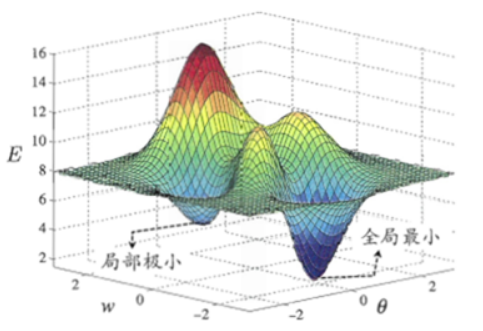• 局部极小解：参数空间中的某个点，其邻域点的误差函数值均不小于该点的误差函数值。
• 全局最小解：参数空间中的某个点，所有其他点的误差函数值均不小于该点的误差函数值。

跳出局部最小的方法：

• 以多组不同参数值初始化多个神经网络，按标准方法训练，迭代停止后，取其中误差最小的解作为最终参数。
• 使用“模拟退火”技术
• 使用随机梯度下降，即在计算梯度时加入了随机因素，使得在局部最小时，计算的梯度仍可能不为0，从而迭代可以继续进行。

## 三、总结：

神经网络可以用作分类、聚类、预测等。神经网络需要有一定量的历史数据，通过历史数据的训练，网络可以学习到数据中隐含的知识。在你的问题中，首先要找到某些问题的一-些特征，以及对应的评价数据，用这些数据来训练神经网络。
虽然BP网络得到了广泛的应用，但自身也存在一- 些缺陷和不足，主要包括以下三个方面的问题。

• 首先，由于学习速率是固定的，因此网络的收敛速度慢，需要较长的训练时间。对于一-些复杂问题，BP算法需要的训练时间可能非常长，这主要是由于学习速率太小造成的，可采用变化的学习速率或自适应的学习速率加以改进。

• 其次，BP算法可以使权值收敛到某个值，但并不保证其为误差平面的全局最小值，这是因为采用梯度下降法可能产生-一个局部最小值。对于这个问题，可以采用附加动量法来解决。

• 再次，网络隐含层的层数和单元数的选择尚无理论上的指导，-般是根据经验或者通过反复实验确定。因此，网络往往存在很大的冗余性，在一定程度上也增加了网络学习的负担。

• 最后，网络的学习和记忆具有不稳定性。也就是说，如果增加了学习样本，训练好的网络就需要从头开始训练，对于以前的权值和阈值是没有记忆的。但是可以将预测、分类或聚类做的比较好的权值保存。

(1)BP神经网络训练。该过程主要利用原始数据集进行BP神经网络的训练，最终形成特定的具有预测功能的神经网络，训练完成后将神经网络保存为net;
该算法的具体流程(训练)如下: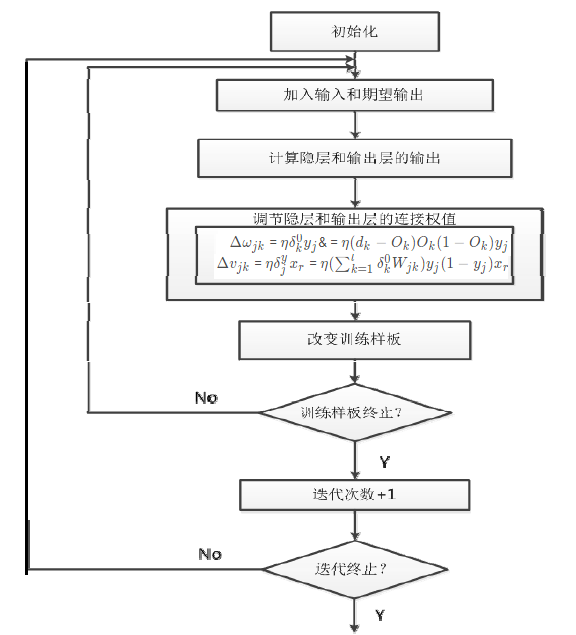(2)利用BP神经网络进行预测。该过程只需要将先前训练好的神经网络载入即可，然后同样对数据进行归一化等操作处理后，即可运行BP神经网络算法，产生一组概率向量。.
影响BP神经网络性能的参数主要有:隐含层节点的个数、激活函数的选择以及学习率的选择等。隐层神经元的个数越少，BP神经网络模拟的效果越差，隐层神经元个数越多模拟的效果越好，然而会使得训练较慢。激活函数对于识别率和收敛速度都有显著的影响，在逼近高次曲线时，S形函数精度比线性函数要高得多，但另一方面计算量也要大得多。学习率直接影响网络的收敛速度，以及网络是否能够收敛。学习率设置偏低虽然能够保证网络收敛，但是收敛速度会很慢，反之如果学习率设置偏高，可能直接导致网络无法收敛，进而降低识别率。

这篇文章就到这里了，欢迎大佬们多批评指正，也欢迎大家积极评论多多交流。### 参考文章：

1 深入理解BP神经网络
2 机器学习——前馈神经网络
3 论文：链接：https://pan.baidu.com/s/1cmn_6GWGvQzBcsNamHA8kQ 提取码：vlix

展开全文• Graph Neural Networks: A Review of Methods and ...物理系统的建模、分子指纹的学习、蛋白质界面的预测和疾病的分类都需要模型从图形输入中学习。在其他领域，如文本、图像等非结构数据的学习中，提取结...
• ## 神经网络学习 之 BP神经网络

万次阅读 多人点赞 2015-11-30 21:17:56
上一次我们讲了M-P模型，它实际上就是对单个神经元的一种建模，...本文具体来介绍一下一种非常常见的神经网络模型——反向传播(Back Propagation)神经网络。概述BP（Back Propagation）神经网络是1986年由Rumelhart和Mc
• 一、学习率衰减的概念和必要性学习率过大，在算法优化的前期会加速学习，使得模型更容易接近局部或全局最优解。但是在后期会有较大波动，甚至出现损失函数的值围绕最小值徘徊，波动很大，始终难以达到最优，如下图...
• BP神经网络代码实现1. BP神经网络的简介和结构参数1.1 BP神经网络的结构组成1.2 BP神经网络训练界面的参数解读 非常感谢博主wishes61的分享. 1. BP神经网络的简介和结构参数 一种按照误差逆向传播算法训练的多层前馈...
• 前面学习了感知器和自适应线性神经网络。 下面介绍经典的三层神经网络结构，其中每个神经元的激活函数采用Sigmoid。PS：不同的应用场景，神经网络的结构要有针对性的设计，这里仅仅是为了推导算法和计算方便才采用...
• 特点是：信号前向传播，误差...通俗理解就是，BP神经网络通过层与层向前传播，得到最终实际输出后，与期望输出做对比，通过“梯度下降”策略，逐层调节权重和阈值，最终得到与期望输出在误差允许范围内的神经网络模型。
• BP神经网络前面我们所讲的几节都是线性神经网络，都无法解决线性不可分的问题，今天我们就来学习非常非常经典的非线性多层前向网络——误差反向传播网络（BP——Error Back Propagtion）。BP神经网络和前面所说的...matlab
• 线性神经网络线性神经网络和单层感知机非常相似，输入层、输出层甚至是误差迭代函数都相同，唯一的区别就是他们的传输函数不同。matlab 线性拟合 人工智能
• 如果输入层有7个节点，输出层1个节点，那么隐含层可暂设为5个节点，即构成一个7-5-1 BP神经网络模型。在系统训练时，实际还要对不同的隐层节点数4、5、6个分别进行比较，最后确定出最合理的网络结构。②、初始权值的...
• 前向网络神经网络分为从传播来讲分为两种： 1.前馈神经网络（前向网络） 2.反馈神经网络前向网络没有反馈机制，也就是自能向前传播而不能反向传播来调整权值参数。感知机就属于前向网络。如上图 一个输入层，一个...matlab 感知机 线性分类
• 训练函数和学习函数是两个不同的函数 ，网络设置中两个都有。简单的说，训练函数确定调整的大算法，是全局调整权值和阈值，考虑的是整体误差的最小； 学习函数决定调整量怎么确定，是局部调整权值和阈值，考虑的是...
• 神经网络学习小记录53——TF2搭建孪生神经网络比较图片相似性（tensorflow2）学习前言什么是孪生神经网络孪生神经网络的实现思路一、预测部分1、主干网络介绍2、比较网络二、训练部分1、数据集的格式2、Loss计算训练...Siamese 卷积
• 神经网络学习小记录24——卷积神经网络经典模型及其改进点汇总学习前言经典神经网络的改进点经典神经网络的结构汇总1、VGG162、ResNet503、InceptionV34、Xception5、MobileNet 学习前言 学了好多好多的网络，它们的...
• 今天要介绍的是常用的RBF神经网络学习算法及RBF神经网络与多层感知器网络的对比。 一、RBF神经网络学习算法 广义的RBF神经网络结构如下图所示： N-M-L结构对应着N维输入，M个数据中心点centers，L个输出。 RBF ...K-means聚类 多层感知器
• 神经网络学习小记录57——Batch Normalization层的原理与作用学习前言什么是Batch NormalizationBatch Normalization的计算公式Bn层的好处为什么要引入γ和β变量Bn层的代码实现 学习前言 Batch Normalization是神经...标准化
• 神经网络学习小记录44——训练资源汇总贴前言权值文件1、迁移学习（传统神经网络）2、目标检测3、实例分割4、语义分割数据集1、迁移学习（传统神经网络）2、目标检测3、实例分割4、语义分割 前言 包含我所有教程内所...
• 一组连接（类比于生物神经元的树突），更准确的讲是一组输入。而每一个输入都会有一个权重进行作用。第k个神经元的第j个树突接收到的一个输入xj，那么这个输入将被权重wkj作用，其中下标表示第k个神经元的第j个树突...
• ## 神经网络学习笔记

万次阅读 2016-04-14 22:41:51
神经网络sigmoid函数sigmoid函数是一种常见的挤压函数，其将较大范围的输入挤压到(0,1)(0,1)区间内，其函数的表达式与形状如下图所示：该函数常被用于分类模型，因为其具有很好的一个特性f′(x)=f(x)(1−f(x))f'(x) ...
• 神经网络学习-MP模型 神经网络学习-MP模型 神经网络学习 之 M-P模型 此链接介绍了mp和感知器人工智能
• 神经网络学习小记录1——利用tensorflow构建卷积神经网络（CNN）学习前言简介隐含层介绍1、卷积层2、池化层3、全连接层具体实现代码卷积层、池化层与全连接层实现代码全部代码 学习前言 学习神经网络已经有一段时间...tensorflow 人工智能
• ## MATLAB神经网络学习

千次阅读 2015-08-05 22:10:13
在这里做一下笔记，来记录matlab神经网络学习过程。以下内容中还有很多是从网上借鉴而来，权当学习。 1.什么是神经网络 比较粗浅的解释是，我们把输入信号当做matlab中需要输入的数据，将这些数据输入到神经网路后，...
• ## 小波神经网络学习程序

千次阅读 多人点赞 2019-01-21 11:21:46
下面来介绍一下MATLAB中的小波神经网络算法的实现过程： 1、用Mexihat函数作为样本输入和输出； 2、数据采用逐个处理，三层网络拓扑结构； 3、赋予网络学习速率和动量因子初始值； 4、初始化输出层和隐层的连接...MATLAB
• 神经网络学习小记录28——深度卷积神经网络各种改进结构块汇总学习前言1、残差网络2、不同大小卷积核并行卷积3、利用(1,x),(x,1)卷积代替(x,x)卷积4、采用瓶颈(Bottleneck)结构5、深度可分离卷积6、改进版深度可分离...
• RBF神经网络是为了解决大部分基于反向传播的多层前馈网络的学习算法必须基于某种非线性优化技术的缺点 ,计算量大、学习速度慢的问题。RBF通过使用高斯和函数的方法，使得，网络由输入到输出的映射是非线性的，而网络...高斯核函数
• 神经网络学习（2）：P，FF，RBF，DFF，RNN，LSTM，CNN…… THE NEURAL NETWORK ZOO 其中大多数是神经网络，但也有些完全不同。此列表并不全面，仍需不断更新。 将它们绘制为节点映射的一个问题是：它并没有......